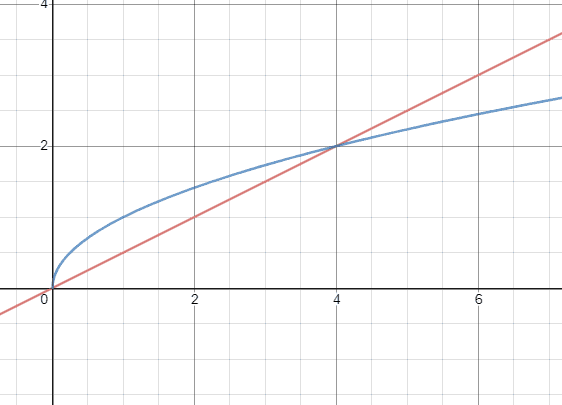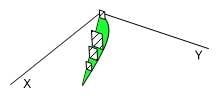# Calculating the volume of the solid in this graph

Homework Statement:
Base of solid is the region bounded by graphs ##y= \sqrt x## and ##y=x/2##. The cross sections perpendicular to the x axis are squares whose sides run across the base of the solid. Find volume of solid.
Relevant Equations:
-
Homework Statement: Base of solid is the region bounded by graphs ##y= \sqrt x## and ##y=x/2##. The cross sections perpendicular to the x axis are squares whose sides run across the base of the solid. Find volume of solid.
Homework Equations: -

As stated above, I will want to calculate the coordinates of the two graphs intersecting, and found them to be x=0 and x=4. However, the equation ## \pi \int_{0}^{4} (\sqrt x - x/2) ^2##seems to be wrong. Referring to my answer key, the answer was simply## \int_{0}^{4} (\sqrt x - x/2)^2## without the pi. Why is this so? Thanks

Mark44
Mentor
Homework Statement: Base of solid is the region bounded by graphs ##y= \sqrt x## and ##y=x/2##. The cross sections perpendicular to the x axis are squares whose sides run across the base of the solid. Find volume of solid.
Homework Equations: -

As stated above, I will want to calculate the coordinates of the two graphs intersecting, and found them to be x=0 and x=4. However, the equation ## \pi \int_{0}^{4} (\sqrt x - x/2) ^2##seems to be wrong. Referring to my answer key, the answer was simply## \int_{0}^{4} (\sqrt x - x/2)^2## without the pi. Why is this so? Thanks
In the future, please post problems that involve derivatives, integrals, and other calculus topics in the Calculus & Beyond section, not in the Precalc section. I will move this thread.

Regarding the problem, why do you have ##\pi## in your integrals? Nothing is being revolved around any axis -- the problem is just a geometric object whose base is a sort of crescent shape.

Draw a sketch of the region, and another sketch of the solid with a few of the vertical slices. Your textbook might even have a drawing of the object, or at least show one or two of the vertical cross sections.

Last edited:
In the future, please post problems that involve derivatives, integrals, and other calculus topics in the Calculus & Beyond section, not in the Precalc section. I will move this thread.

Regarding the problem, why do you have ##\pi## in your integrals? Nothing is being revolved around any axis -- the problem is just a geometric object whose base is a sort of crescent shape.

Draw a sketch of the region, and another sketch of the solid with a few of the vertical slices. Your textbook might even have a drawing of the object, or at least show one or two of the vertical cross sections.
Sorry. I will take note of this in the future.

As for the question, I don't really understand what the question means by:" The cross-sections perpendicular to the x axis are squares whose sides run across the base of the solid. Find the volume of solid. "

Sketching the graphs as shown:Am I just supposed to find the following shaded area in green? What about the second sentence?Mark44
Mentor
Am I just supposed to find the following shaded area in green?
No, you're supposed to find the volume of the solid whose base is the green region.•